# # MatLab

## # Prerequisites

### # Install MatLab

• Install MatLab `R2017b` with Database Toolbox

To complete this exercise, sample data must be available in your ATSD instance.

2. Open Metrics > Data Entry, open the Commands tab.
3. Copy the `series` commands into the form and click Submit.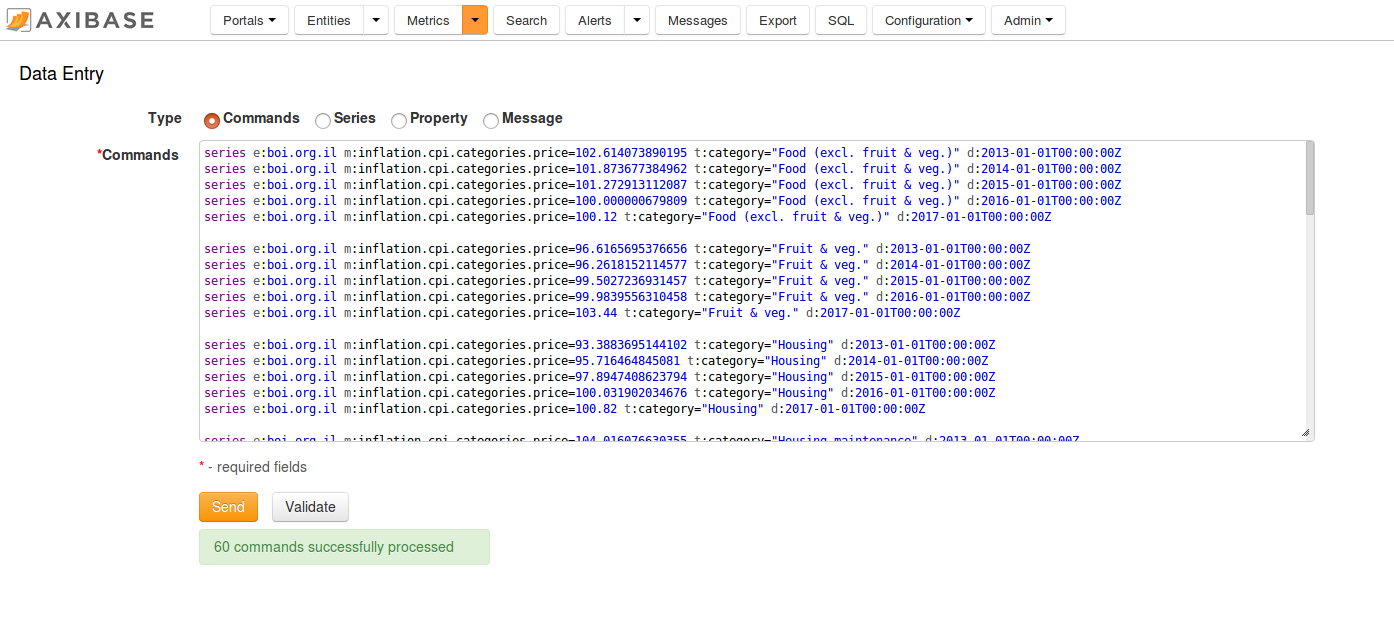The commands contain the Consumer Price Index (CPI) for each category of items in a consumer basket as well as a weight for each category in the CPI basket. The weights are stored as fractions of 1000. The CPI is tracked from 2013 to 2017 and uses Year 2016 values as the baseline. Weight values are available only for 2017. The underlying data is available in the following Excel file.

To calculate a weighted inflation index we need to multiply the CPI of each category by its weight divided by 1000 and sum the products.

### # Install ATSD Driver

There are two ways of enabling the ATSD JDBC driver in MatLab: static and dynamic

#### # Static

• Run the `prefdir` command in the MatLab Command Window. This command displays the path to a directory used in subsequent steps.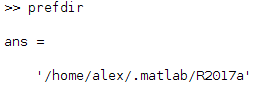• Close MatLab if the application is running.
• Change to the `prefdir` directory above and create a file named `javaclasspath.txt`.
• Open the `javaclasspath.txt` file. Add the full path to the ATSD driver JAR file, for example, `/home/user/MATLAB/atsd-jdbc-1.2.22-DEPS.jar`
• Save and close `javaclasspath.txt`.
• Restart MatLab.

#### # Dynamic

• Run the `javaaddpath('dpath')` command in the MatLab Command Window, where `dpath` is the full path to the ATSD driver JAR file.

Example: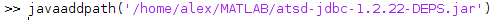## # Configure Database Connection

### # Connect in Database Explorer

• Apps > Database Explorer
• Open Configure Data Source > Configure JDBC Data Source
• Select Vendor > OTHER.
• Set the Driver field to `com.axibase.tsd.driver.jdbc.AtsdDriver`
• Specify a JDBC URL, for example `jdbc:atsd://atsd_hostname:8443;expandTags=true` Information about ATSD JDBC URL parameters
• If connection is successful click Save and close JDBC Data Source Configuration window

Example: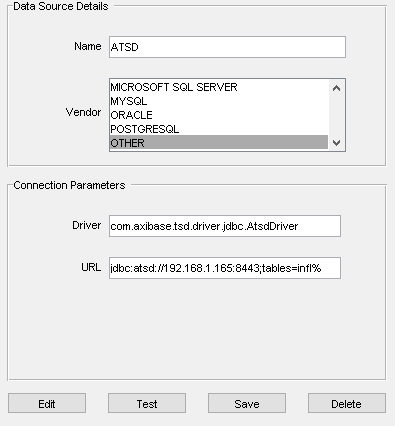`atsd_hostname` is the hostname address of the ATSD instance you want to connect to.

### # Connect to the MatLab Command Window

Example of HTTPS connection to ATSD:

``````driver = 'com.axibase.tsd.driver.jdbc.AtsdDriver';
url = 'jdbc:atsd://atsd_hostname:8443';
``````

## # Verify Connection

ATSD connection to Command Window can be verified using the `SELECT 1` query. Assuming `conn_atsd` is defined:

``````sqlquery = 'SELECT 1';
curs = exec(conn_atsd, sqlquery);
res = fetch(curs);
data = res.Data
``````

Expected result: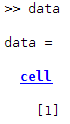## # Review Tables in the Database Explorer

• Specify a JDBC URL, for example `jdbc:atsd://atsd_hostname:8443;tables=%jvm%` in the JDBC Data Source Configuration window.
• Click New Query, select ATSD in the Data Source drop-down list, enter ATSD username and password.

The Database Browser displays a set of tables matching the expression: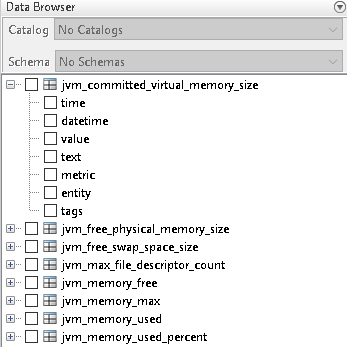Select one or more columns to open the Data Preview window displaying the first `N` rows of the result set (10 by default).

An example with the `datetime`, `value`, and `text` fields selected: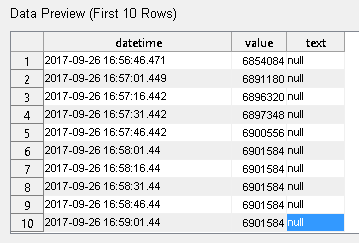The complete result set can be imported with the Import button, highlighted with a green arrow.

Execute the SQL query to import the result set into a MatLab variable:

``````sqlquery = "SELECT * FROM gc_time_percent LIMIT 50";
curs = exec(conn_atsd, sqlquery);
res = fetch(curs);
data = res.Data;
``````

## # Write Data

`data` example: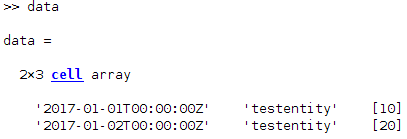To insert data into ATSD (assuming the `data` variable has required values):

``````colnames = {'datetime', 'entity', 'value'};
insert(conn_atsd, 'METRIC_NAME', colnames, data);
``````

`colnames` is a cell array which describes the names and order of columns in the request.

`METRIC_NAME` is the name of a metric under which the rows in `data` are inserted.

## # Calculate Derived Series

### # Connect to ATSD

``````driver = 'com.axibase.tsd.driver.jdbc.AtsdDriver';
url = 'jdbc:atsd://atsd_hostname:8443';
``````

Load pricing data into the variable `prices`

``````% SQL query to get prices for a date range
sqlquery = "SELECT datetime, tags.category, value FROM inflation.cpi.categories.price WHERE datetime BETWEEN '2013-01-01T00:00:00Z' AND '2017-01-01T00:00:00Z' ORDER BY 1, 2";
% get cursor from ATSD
curs = exec(conn_atsd, sqlquery);
% fetch data from cursor
res = fetch(curs);
% initialize result set from data as cell array
prices_resultset = res.Data;
``````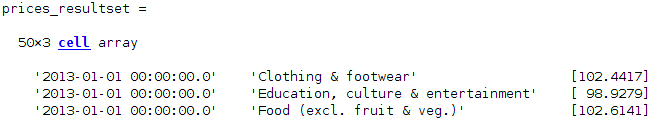``````% fetch datetime column
datetimes = prices_resultset(:,1);
``````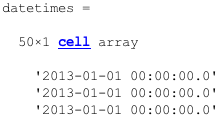``````% get every 10th record from datetimes to form list of years
datetimes = datetimes(1:10:length(datetimes));
``````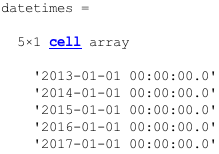``````% fetch third column from prices result set (value field)
% convert column to numeric array
prices = cell2mat(prices_resultset(:,3));
``````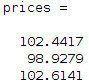Load weights data into the variable `weights`

``````% SQL query to get weights
sqlquery = "SELECT tags.category, value FROM inflation.cpi.categories.weight WHERE datetime = '2017-01-01T00:00:00Z' ORDER BY 1";
% get cursor from ATSD
curs = exec(conn_atsd, sqlquery);
% fetch data from cursor
res = fetch(curs);
% initialize weights result set from data as cell array
weights_resultset = res.Data;
% fetch second column from weights result set (value field)
% convert column to numeric array
weights = cell2mat(weights_resultset(:,2));
% repeat weights column for 2017 values (5,1 means that resulting matrix increases in height 5 times)
weights = repmat(weights, 5, 1);
``````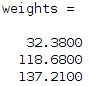### # Calculate Weighted Index

``````% element-wise multiply of 2 columns (1000 value is here because weights is a proportion out of 1000)
inflation_cpi_price = prices .* weights / 1000;
``````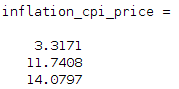``````% sum inflation prices for each year
inflation_cpi_composite_price = sum(reshape(inflation_cpi_price, 10, 5));
% transponse vector (transform row to column)
inflation_cpi_composite_price = inflation_cpi_composite_price';
``````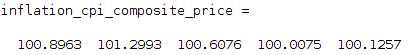### # Create cell-matrix to insert into ATSD

``````% form list of entities for result payload
entity = 'bls.gov';
entities = repmat(cellstr(entity), size(datetimes, 1), 1);
``````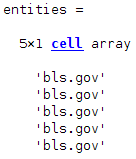``````% append Entity, Datetime and Inflation columns
``````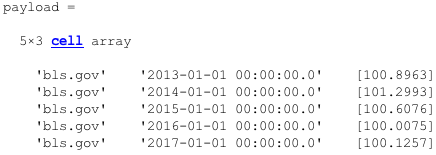``````% define colnames which is a cell array describing names and order of columns in payload
colnames = {'entity', 'datetime', 'value'};
% insert data into ATSD